# Slope of polyline - Python syntax error 000989

2734
10
03-09-2016 07:25 AMNew Contributor III

Hi

I am trying to calculate the % slope of multiple polylines in a shapefile. I am using Arc 10.1 with the basic licence.

When I run my code in the Field Calculator I get a Python syntax error on line 3. I can't see the issue - can someone tell me where I'm going wrong?

My code is: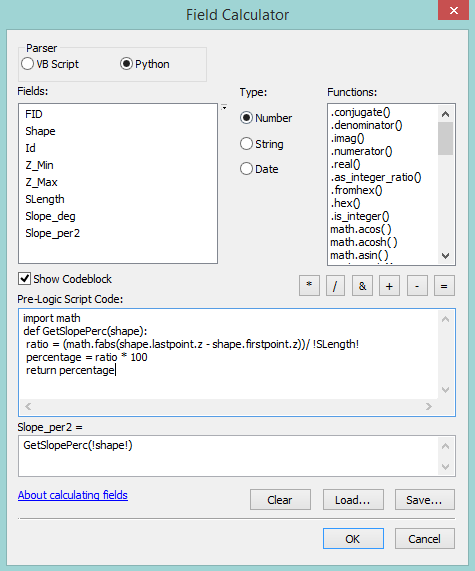NB - I am not sure what directions the polylines were digitised in. Hence I don't know if the last point is at the higher elevation or vice versa, which is why I am trying to return the absolute change in z value.

The error read out I am getting when I run the code is: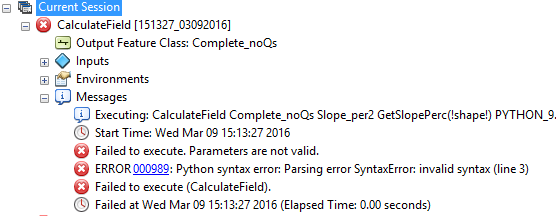1 Solution

Accepted SolutionsNew Contributor III

I've written up the solution to this problem, including Field Calculator Python codes for straight line length, percentage slope and degrees slope. See this document for my write-up:

10 RepliesbyMVP Esteemed Contributor

in your expression box, it should be !Shape! with the s capitalized.  Also check your indentation, it looks sketchy.  And you need to pass the !Slength field in as wellNew Contributor III

I've made changes to the expression box and the indentation. What do you mean about passing the SLength field in?

At the moment I'm still getting the same error, with the syntax issue still on line 3.

Here is the code:New Contributor III

Just noticed the error on line 4 in the above code. Have corrected it, but it wasn't the cause of the issue.byMVP Esteemed Contributor

GetSlopePerc(!Shape!,!SLength!)

def GetSlopePerc(Shape,Leng)

in ration change !SLength! to LengNew Contributor III

Hi again. I've only just had the chance to test these changes but the code is still returning an error.

Here is my current code: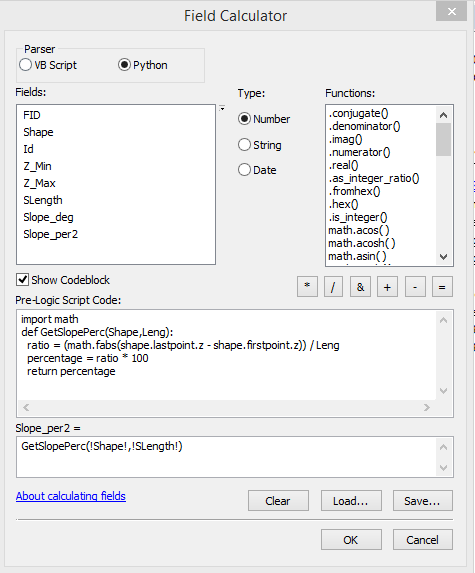This is the error that it is returning: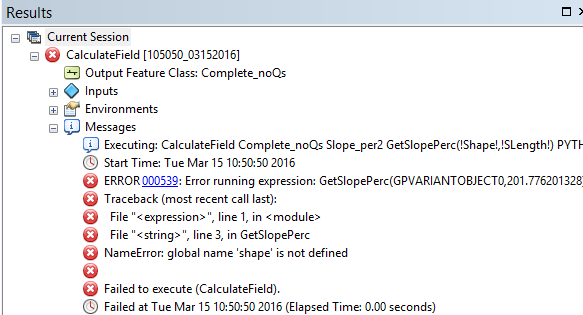Thanks for your other point about the straight line slope. On thinking about it I do think that I will need to use the straight line slope. So I will add another field to compute the straight line distance between the first and last points and will substitute this field for SLength.New Contributor III

I've resolved the NameError and the code is now executing. I have also computed the straight line length of the polylines sucessfully and substituted this field into the code in plac of SLength. This is my current code: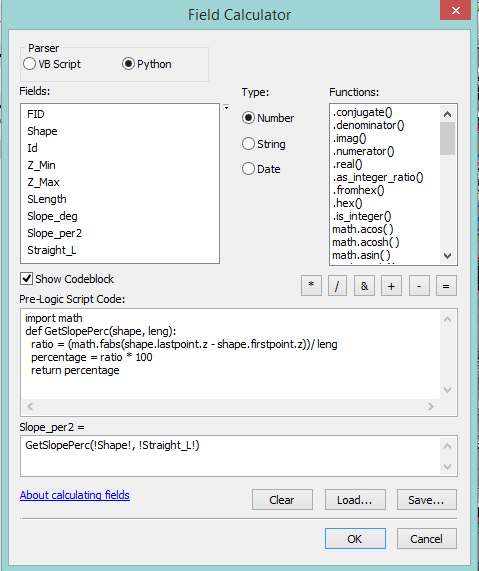At the moment this is only returning a value of 0. Working through the calculation for a couple of the polylines suggests that I should be getting values other than 0. Do you have any idea why I am only getting 0 values out of this code?byMVP Esteemed Contributor

Your z values are the same.  I would calculate the value of the first and last point using Add Geometry Attributes—Help | ArcGIS for Desktop

and you could use the z values from the resultant tableNew Contributor III

I've got it working.

Unfortunately my university is tight and will only supply me with Arc 10.1, which doesn't have the Add Geometry Attributes tool. So I used the old fashioned 'Calculate geometry' function to add start and end z values to the attribute table. However, this was returning a z value of 0 for both the start and end points of every polyline.

After a bit of snooping I discovered that the z values for the layer had somehow reset to 0. Presumably during some other processing I'd done previously. So I re-extracted correct z values from a DEM of my study area. This got 'calculate geometry' to return the right z values and meant that my slope codes worked perfectly.

Here is the final code for percentage slope (using straight line length between the start and end points):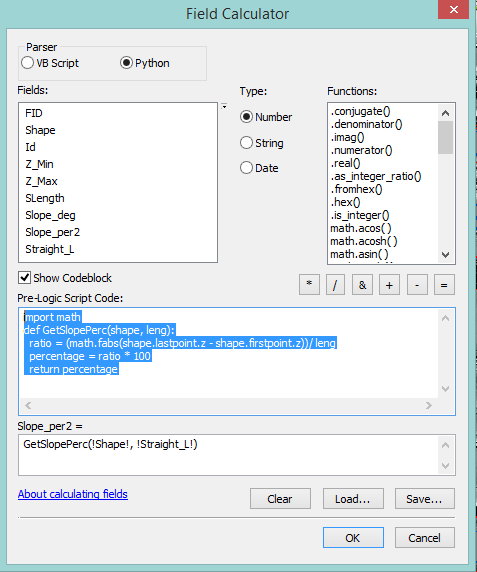And here is the code I used to get slope in degrees from the percentage: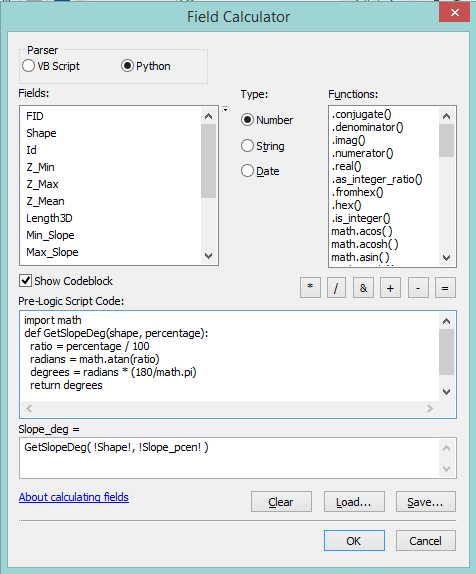by